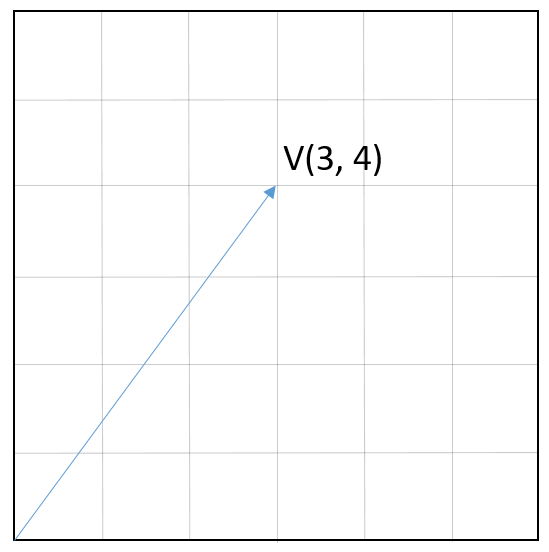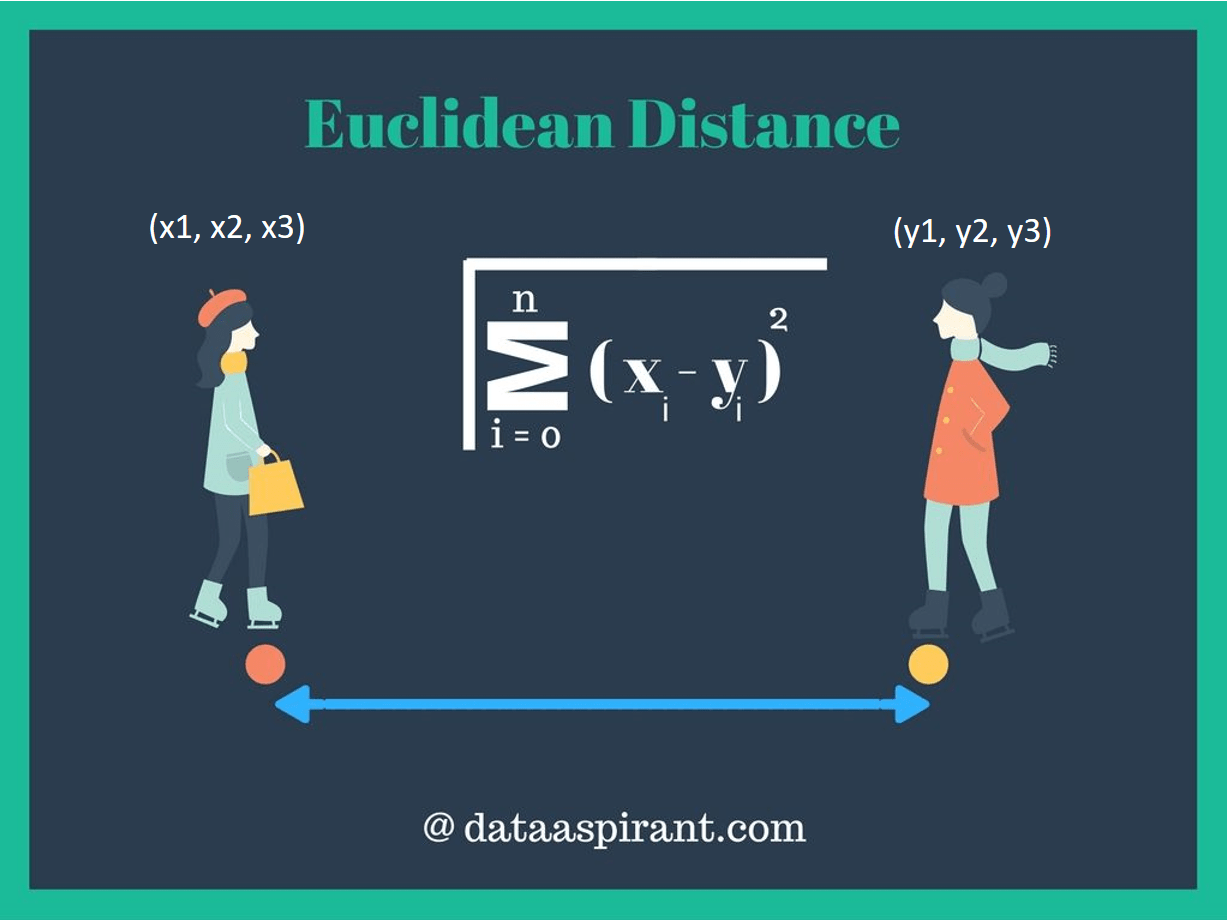# Cosine Similarity in Machine Learning

Haven't you subscribe my channel yet?

Follow me on Twitter

Some machine learning tasks such as face recognition or intent classification from texts for chatbots requires to find similarities between two vectors. Herein, cosine similarity is one of the most common metric to understand how similar two vectors are. In this post, we are going to mention the mathematical background of this metric.Find the different one

### A Poem

Let’s start with a poem by Turkish poet Can YucelThe longest distance is neither Africa nor China or India, Nor planets or stars shining at nights. It is between two minds that don’t understand each other

So, could euclidean distance find the distance between two minds that don’t understand each other?

### Dot product

Dot product is a way to multiply vector. This approach produces scalar results. Let a and b be vectors.

a = ( a1, a2, …, an)

b = (b1, b2, …, bn)

Definition of dot product state adding multiplication of same index items of a and b.

a . b = a1b1 + a2b2 + … + anbn

If a and b stored as a column vector, then multiplying transposed version of a and b give same result. Notice that matrix operations can be handled much faster than for loops.

a . b = ab

### Law of cosine

Let a and b be vectors and theta be the angle between these vectors.Sample vectors

Let’s define a new vector c which is equal to a – b (or -a+b). As seen, a, b and c vectors create a valid triangle whereas vector c can be expressed as (a-b).Creating a new vector to draw a triangle

Herein, law of cosines states

||c||2 = ||a||2 + ||b||2 – 2||a|| ||b|| cosθ

where ||a||, ||b|| and ||c|| denote vector length of a, b and c respectively.

Remember that vector c is equal to a – b.

||c||2 = c.c = (a-b)(a-b) = a.a – a.b – b.a + b.b = ||a||2 + ||b||2 – a.b – b.a

Notice that -a.b and -b.a are equal to each other because they are dot products. Please remember these terms are scalar, not vectors.

We can rearrange the length of vector c squared as

||c||2 = ||a||2 + ||b||2 – 2 a.b

Let’s compare the law of cosine and this term.

||c||2 = ||a||2 + ||b||2 – 2||a|| ||b|| cosθ = ||a||2 + ||b||2 – 2 a.b

The only difference is that one equation is expressed as length of vectors and angle between them, and another equation is expressed as dot product.

– 2||a|| ||b|| cosθ = – 2 a.b

We can divide both side of equation to minus 2.

a.b = ||a|| ||b|| cosθ

Recall the definition of dot product.

a1b1 + a2b2 + … + anbn = ||a|| ||b|| cosθ

I wonder the cosine theta term

cosθ = (a1b1 + a2b2 + … + anbn) / ||a|| ||b||

Well, how to calculate the length of a vector?

### Vector Length

Finding length of a vector is an easy task. Let V be a vector on a 2D space and (V1 = 3, V2 = 4). As you guess, length of this vector is 5. It originally comes from Pythagorean theorem.Finding vector length

Logic remains same for n-dimensional space. Formula of vector length calculation is shown below.

||V|| = √(∑ (i = 1 to n) Vi2)

### Putting all those ideas together

Let a and b be vectors. Similarity formulation of these two vectors can be generalized as mentioned below.

cosine similarity = (a1b1 + a2b2 + … + anbn) / (√(∑ (i = 1 to n) ai2) √(∑ (i = 1 to n) bi2))

or we can apply vectorization to find cosine similarity

cosine similarity = (ab) / (√(aa) √(bb))

In this way, similar vectors will produce high results.

### Cosine distance

Distance between similar vectors should be low. We can find the distance as 1 minus similarity. In this way, similar vectors should have low distance (e.g. < 0.20)

cosine distance = 1 – cosine similarity

### Code wins arguments

We can adapt cosine similarity / distance calculation into python easily as illustared below.

```def findCosineDistance(vector_1, vector_2):
a = np.matmul(np.transpose(vector_1), vector_2)

b = np.matmul(np.transpose(vector_1), vector_1)
c = np.matmul(np.transpose(vector_2), vector_2)

return 1 - (a / (np.sqrt(b) * np.sqrt(c)))
```

### An Alternative: Euclidean Distance

Cosine similarity is not the only metric to compare vectors. Remember that vectors are objects has length and direction. If the length of the vector were not important for your task, then cosine similarity works well because it only matters the angle between vectors. I mean that if you have similar vectors such as (3, 4) and (6, 8), then these vectors are exactly similar based on cosine similarity. However, these vectors also have length. Length of first one is 5 and length of second one is 10 based on Pythagorean theorem. We cannot say that these vectors are same. Distance between these two vectors is 5. This is euclidean distance.Euclidean distance by dataaspirant

Euclidean distance = √(∑(i=0 to n) (ai – bi)2 )

where a and b are vectors and n refers to dimensions.

We can adapt euclidean distance in python from scratch.

```
def findEuclideanDistance(a, b):
euclidean_distance = a - b
euclidean_distance = np.sum(np.multiply(euclidean_distance, euclidean_distance))
euclidean_distance = np.sqrt(euclidean_distance)
return euclidean_distance
```

Applying the correct comparison metric depends on the problem just like error metrics in ML. Remember face recognition tasks. Herein, I prefer to check cosine similarity because length of two vectors are not important. I just wonder how similar two vectors are. But nobody objects you if you use euclidean distance instead of cosine similarity.

So, we have mentioned the theoretical background of cosine similarity in this post. This metric is mainly based on law of cosines. It produces efficient results so fast to understand how similar two vectors are.

Like this blog? Support on PatreonThis site uses Akismet to reduce spam. Learn how your comment data is processed.